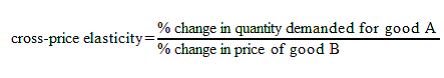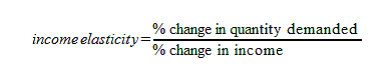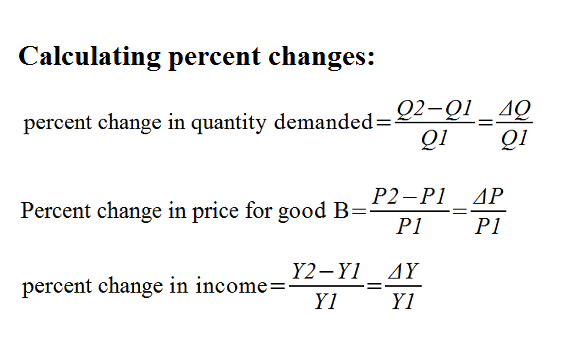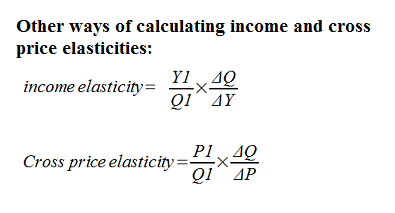Wednesday, March 7, 2012

Income and Cross-Price Elasticities of Demand

In regular economics class, we have learned that buyers’ incomes and prices of other goods are both demand determinants that shift the demand curve. Now, income elasticity and cross-price elasticity are measures that tell us “how much” the demand is shifted. In other words, income elasticity of demand gives us information about how responsive the demand (for a good) is to a change in income . Cross-price elasticity tells us how responsive the demand (for a good) is to a change in the price of another good.

Income Elasticity:
• Definition: the measure of how much the demand for one good responds to a change in consumers’ income.
• How to calculate: see “formulas”
• Other important information:
• A good is inferior if it has a negative income elasticity
• A good is normal if it has a positive income elasticity
• The greater the absolute value of the income elasticity, the more income-elastic the good is (the more the demand is affected by the income)
• If income elasticity is between zero and one, the good is income-inelastic, and can be classified as a normal necessity
• If income elasticity >1, the good is income- elastic, and can be classified as a luxury good.

Cross-price elasticity:
• Definition: the measure of how much the demand for one good responds to a change in the price of another good.
• How to calculate: see “formulas”
• Other important information:
• Two goods are complements if they have a negative cross-price elasticity
• Two goods are substitutes if they have a positive cross-price elasticity
• The larger the absolute value of the cross-price elasticity, the stronger the two goods are related to each other

Formulas (first two are essential, others are not necessary, but good to know):